# Generalized Linear Models II¶

Nov. 25, 2018 $\newcommand{\bs}{\boldsymbol}$ $\newcommand{\argmin}{\underset{\bs{#1}}{\text{arg min}}\,}$ $\newcommand{\argmax}{\underset{\bs{#1}}{\text{arg max}}\,}$ $\newcommand{\tr}{^{\top}}$ $\newcommand{\norm}{\left|\left|\,#1\,\right|\right|}$ $\newcommand{\given}{\,|\,}$ $\DeclareMathOperator{\E}{\mathbb{E}}$ $\newcommand{\blue}{{\color{blue} {#1}}}$ $\newcommand{\red}{{\color{red} {#1}}}$ $\newcommand{\orange}{{\color{orange} {#1}}}$ $\newcommand{\pfrac}{\frac{\partial #1}{\partial #2}}$

Recall that the data model for the generalized linear model has the form

$$p(y_i \given \theta_i) = \text{exp} \left(\frac{y_i\theta_i-b(\theta_i)}{a_i(\phi)} + c(y_i, \phi) \right), \tag{1}$$

where the natural parameters $\theta_i$ is related to the regression coefficients $\beta_i$ through

$$\mu_i = b'(\theta_i) = g^{-1}(\eta_i) = g^{-1} \left(\sum_j \beta_j x_{ij}\right), \tag{2}$$

The Bayesian model is complete by the specification of a prior distribution

$$\bs{\beta} \sim \mathcal{N}(\bs{a}, \bs{R}). \tag{3}$$

Hence, for this post, we will consider the posterior distribution

$$\pi(\bs{\beta}) \propto \text{exp} \left(-\frac{1}{2}(\bs{\beta} - \bs{a})\tr \bs{R}^{-1}(\bs{\beta}-\bs{a}) + \sum_{i=1}^n \frac{y_i\theta_i - b(\theta_i)}{a_i(\phi)} \right). \tag{4}$$

Recall the iterative weighted least squares (IWLS) from the previous post with a slight modification:

IWLS Algorithm

• Initialize the parameter $\bs{\beta}^{(0)}$.
• For $t=1,2,...,N$,
• Calculate the estimated linear predictor $\bs{\eta}^{(t-1)} = \bs{X} \bs{\beta}^{(t-1)}$, and the fitted means $\bs{\mu}^{(t-1)} = g^{-1}(\bs{\eta}^{(t-1)})$.
• Using these quantities, we calculate the adjusted response variable $\bs{z}^{(t-1)}$ given by $$z_i^{(t-1)} = \eta_i^{(t-1)} + (y_i - \mu_i^{(t-1)}) g'(\mu_i^{(t-1)}).$$
• We calculate the weights $$w_i = \left[b''(\theta_i) \left(g'(\mu_i^{(t-1)})\right)^2 a_i(\phi)\right]^{-1}$$
• We obtain $\bs{\beta}^{(t)}$ as the WLS estimator of $\bs{\beta}$ from the weighted least squares model $$\bs{z}^{(t-1)} \sim \mathcal{N}(\bs{X}\bs{\beta}^{(t-1)}, \bs{W}^{-1}).$$
• The WLS estimator is given by, $$\bs{\beta}^{(t)} \leftarrow (\bs{X}\tr \bs{W} \bs{X})^{-1}\bs{X}\tr \bs{W}\bs{z}^{(t-1)}.$$
• In addition, we can obtain the asymptotic covariance matrix of the WLS estimator as the inverse of the Fisher information matrix $\mathcal{I}$ given by $$\bs{C}^{(t)} \leftarrow (\bs{X}\tr \bs{W} \bs{X})^{-1}. \tag{5}$$
• The procedure is repeated until convergence.

## Bayesian IWLS¶

The Bayesian version of the IWLS algorithm is inspired by the frequentist version with the addition of a prior distribution $\mathcal{N}(\bs{a}, \bs{R})$ for $\bs{\beta}$. Instead of solving a weight least squares problem at each iteration, the Bayesian algorithm obtains a draw of $\bs{\beta}$ from the posterior distribution $\mathcal{N}(\bs{\beta}^{(t)}, \bs{C}^{(t)})$ obtained from using the prior $\mathcal{N}(\bs{a}, \bs{R})$ for $\bs{\beta}$ and the prior $\mathcal{N}(\bs{X}\bs{\beta}^{(t-1)}, \bs{W}^{-1})$ for the adjusted response $\bs{z}^{(t-1)}$. The posterior has the form

\begin{aligned} \pi(\bs{\beta}) &\propto \text{exp}\left\{-\frac{1}{2}\left(\bs{z}-\bs{X}\bs{\beta})\tr\bs{W} (\bs{z}-\bs{X}\bs{\beta}) + (\bs{\beta}-\bs{a})\tr\bs{R}^{-1}(\bs{\beta}-\bs{a})\right) \right\} \\ & \propto \text{exp}\left\{-\frac{1}{2}\left(\bs{\beta}\tr (\bs{X}\tr\bs{W}\bs{X}+\bs{R}^{-1})\bs{\beta} -2 (\bs{z}\tr\bs{W}\bs{X} + \bs{a}\tr\bs{R}^{-1})\bs{\beta}\right)\right\}. \end{aligned}

Recall the kernel of the multivariable normal distribution $\bs{Z} \sim \mathcal{N}(\bs{\mu}, \bs{\Sigma})$ has the form

$$p(\bs{z}) \propto \text{exp} \left\{-\frac{1}{2}\left(\bs{z}\tr \bs{\Sigma}^{-1}\bs{z} - 2\bs{\mu}\tr \bs{\Sigma}^{-1}\bs{z} \right) \right\}.$$

Comparing these two, we conclude that the parameters of the posterior distribution $\mathcal{N}(\bs{\beta}^{(t)}, \bs{C}^{(t)})$ are

\begin{aligned} \bs{\beta}^{(t)} &= \left(\bs{R}^{-1}+\bs{X}\tr\bs{W}\bs{X}\right)^{-1}\left(\bs{R}^{-1}\bs{a}+\bs{X}\tr\bs{W}\bs{z}^{(t-1)}\right) \\ \bs{C}^{(t)} &= \left(\bs{R}^{-1}+\bs{X}\tr\bs{W}\bs{X}\right)^{-1} \end{aligned} \tag{6}

This fits perfectly with the idea of the proposal density in the Metropolis-Hastings algorithm. We have the following sampling scheme.

Bayesian IWLS Sampling

• Initialize the value $\bs{\beta}^{(1)}$.
• for $t=1,...,N-1$:
• Calculate the estimated linear predictor $\bs{\eta}^{(t-1)} = \bs{X} \bs{\beta}^{(t-1)}$, and the fitted means $\bs{\mu}^{(t-1)} = g^{-1}(\bs{\eta}^{(t-1)})$.
• Using these quantities, we calculate the adjusted response variable $\bs{z}^{(t-1)}$ given by $$z_i^{(t-1)} = \eta_i^{(t-1)} + (y_i - \mu_i^{(t-1)}) g'(\mu_i^{(t-1)}).$$
• We calculate the weights $$w_i = \left[b''(\theta_i) \left(\pfrac{\eta_i}{\mu_i}\right)^2 a_i(\phi)\right]^{-1} = \left[\text{Var}(Y_i) \left(g'(\mu_i^{(t-1)})\right)^2 \right]^{-1}$$
• Compute the parameters \begin{aligned} \bs{\beta}^{(t)} &= \left(\bs{R}^{-1}+\bs{X}\tr\bs{W}\bs{X}\right)^{-1}\left(\bs{R}^{-1}\bs{a}+\bs{X}\tr\bs{W}\bs{z}^{(t-1)}\right) \\ \bs{C}^{(t)} &= \left(\bs{R}^{-1}+\bs{X}\tr\bs{W}\bs{X}\right)^{-1} \end{aligned}
• Generate a value $\bs{\beta}^*$ from the proposal density $$\bs{\beta}^* \sim J(\bs{\beta} \given \bs{\beta}^{(t)}) \sim \mathcal{N}(\bs{\beta}^{(t)}, \bs{C}^{(t)}).$$
• We set $\bs{\beta}^{(t+1)} \leftarrow \bs{\beta}^*$ with the acceptance probability equal to $$\alpha=\min\left\{1, \frac{p(\bs{\beta}^* \given \bs{Y})\,J(\bs{\beta}^{(i)} \given \bs{\beta}^*)}{p(\bs{\beta}^{(i)} \given \bs{Y})\,J(\bs{\beta}^* \given \bs{\beta}^{(i)}) }\right\}.$$

The key feature of this algorithm is that the proposal density is a good approximation of the true posterior distribution $\pi$, so this leads to a high acceptance rate without compromising the quality of the chain.

## Logistic Regression¶

The logistic regression proposes the following data model

\begin{aligned} p(y_i) &= \pi_i^{y_i}(1-\pi_i)^{1-y_i} \\ \text{logit}(\pi_i) &= \bs{x}_i\tr \bs{\beta} = \eta_i \end{aligned} \tag{7}

The canonical link function is $g(\mu_i) = \text{logit}(\mu_i)$. Its derivative is given by $g'(\mu_i) = 1/(\mu_i(1-\mu_i))$. Hence, at each iteration of the Bayesian IWLS, the weights $\bs{W} = \text{diag}(w_i)$ is given by

$$w_i = \frac{1}{\text{Var}(Y_i)(g'(\pi_i))^2} = \pi_i(1-\pi_i). \tag{8}$$

Given univariate data $(\bs{y}, \bs{X})$, where $\bs{y} = (y_1,...,y_n)'$ and $\bs{X}=(\bs{x}_1,...,\bs{x}_p)'$, the likelihood function is given by

$$p(\bs{\beta}) = \prod_{i=1}^n P(\eta_i)^{y_i} (1-P(\eta_i))^{1-y_i},$$

where $P(\cdot) = \text{exp}(\cdot)/(1+\text{exp}(\cdot))$ is the logistic function.

Taking the log, we obtain the log-likelihood of $\bs{\beta}$:

\begin{aligned} l(\bs{\beta}) &= \sum_{i=1}^n (y_i (\eta_i-\log(1+\text{exp}(\eta_i)) + (1-y_i)(-\log(1+\text{exp}(\eta_i)) \\ &=\sum_{i=1}^n y_i \eta_i-\log(1+\text{exp}(\eta_i)). \end{aligned}

In R, an efficient way of computing the log likelihood is using the dbinom function:

In :
# define logistic function
P <- function(x){
exp(x)/(1+exp(x))
}

# define log-likelihood for beta
p.log <- function(X, y, beta){
sum(dbinom(y, 1, P(X %*% as.vector(beta)), log=TRUE)) %>% as.vector()
}


For demonstration purposes, let's consider the Pima Indian diabetes data set. The dataset contains observations of 532 patients with 7 predictors and a binary response variable that equals 1 if the patient has diabetes and 0 otherwise. The dataset is included in R's MASS package.

In :
# load necessary packages
library(MASS)
library(mvtnorm)
library(dplyr)

In :
# obtaining dataset
pima <- rbind(Pima.tr, Pima.te)
rownames(pima) <- NULL

npregglubpskinbmipedagetype
5 86 68 28 30.2 0.36424 No
7 195 70 33 25.1 0.16355 Yes
5 77 82 41 35.8 0.15635 No
0 165 76 43 47.9 0.25926 No
0 107 60 25 26.4 0.13323 No
5 97 76 27 35.6 0.37852 Yes
In :
# generate response vector y
y <- pima[,8] %>% as.vector()
y[y=='Yes'] <- 1
y[y=='No'] <- 0
y <- as.numeric(y)

# obtain design matrix with BMI as the only covariate
X <- cbind(1, pima[,5]) %>% as.matrix()

# plotting data
options(repr.plot.width=3, repr.plot.height=3)
par(mar=c(4, 4, 2, 1))
plot(X[,2], y, xlab='BMI', cex=0.5)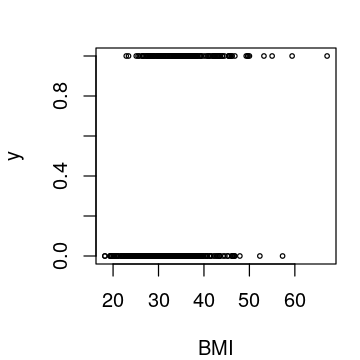For simplicity, we initialize $\bs{\beta}^{(1)} = (0,0)'$, and define a prior distribution $\mathcal{N}(\bs{a}, \bs{R})$, where

\begin{aligned} \bs{a} &= (0,0)' \\ \bs{R} &= \begin{pmatrix} 10 & 0 \\ 0 & 10 \end{pmatrix} \end{aligned}
In :
# initialize the chain
beta0 <- c(0,0)
a <- c(0,0)
R <- 10*diag(2)

In :
# returns mean and variance for proposal density
J.params <- function(X, beta, Ri, Ria){
#     parameters:
#     -----------
#     X = design matrix of data
#     beta = current value of beta in an iteration of IWLS
#     Ri, Ria = R^-1 and R^-1*a

# linear predictor
eta <- X %*% beta %>% as.vector()

# means (P is the logistic function)
mu <- P(eta) %>% as.vector()

z <- eta + (y-mu)/(mu*(1-mu)) %>% as.vector()

# the weight matrix W
W <- diag(mu*(1-mu))

# computing proposal mean and covariance
C.t <- solve(Ri + t(X) %*% W %*% X)
beta.t <- C.t %*% (Ria + t(X) %*% W %*% z)

return(list('beta'=beta.t, 'C'=C.t))
}

In :
# returns the acceptance probability
alpha.calc <- function(beta.new, beta.old, X, y, C) {
#     parameters:
#     -----------
#     beta.new = value of beta generated at current iteration
#     beta.old = value of beta from previous iteration
#     X, y = design matrix and response vector
#     C = covariance matrix of the proposal density
top <- p.log(X,y,beta.new) + dmvnorm(beta.old,beta.new,C,log=TRUE)
bot <- p.log(X,y,beta.old) + dmvnorm(beta.new,beta.old,C,log=TRUE)
min(1, exp(top-bot))
}

In :
# Bayesian IWLS algorithm for logistic regression
logit.mcmc <- function(X, y, N, beta0, a, R, thin=1, burnin=0, seed=6, plot=TRUE) {
#     parameters:
#     -----------
#     X, y = design matrix and response vector
#     N = number of iterations
#     beta0 = initial value for beta
#     a, R = prior parameters for beta
#     thin = skipping iterations to decorrelate the chain
#     burnin = discarding the initial part of the chain
set.seed(seed)
acc = 0 # acceptance

# Initialize beta and create saving device
beta <- beta0
beta.mcmc <- matrix(0, ncol=dim(X), nrow=(N/thin-1))

# compute quantities R^-1 and R^-1*a
Ri <- solve(R)
Ria <- Ri %*% a

for (t in 1:(N-1+burnin)){

# obtain parameters for the proposal density
params <- J.params(X, beta, Ri, Ria)
beta.t <- params$beta %>% as.vector() C.t <- params$C %>% as.matrix()

# generate a new value of beta from the proposal density
beta.s <- rmvnorm(1, beta.t, C.t) %>% as.vector()

# Compute acceptance probability
alpha <- alpha.calc(beta.s, beta, X, y, C.t)

# MCMC Update
R <- rbinom(1,1,alpha)

if (R==1){
beta <- beta.s
acc <- acc+1
}

# Saving the thinned Markov chain
if ((t %% thin == 0) & (t >= burnin)){
beta.mcmc[(t-burnin)/thin,] = as.vector(beta)
}
}

p <- length(beta0)

if (plot==TRUE){

# print basic information
print('SUMMARY')
print('-------------------------', quote=FALSE)
print(paste('Iterations:', N))
print(paste('Burn-in:', burnin))
print(paste('Thin:', thin))
print(paste('Acceptance rate:', acc/N))
print('-------------------------', quote=FALSE)

options(repr.plot.width=6, repr.plot.height=2*p)
par(mfrow=c(p, 1), mar=c(4, 4, 2, 1))

# plot the chains
for (i in 1:p){
plot(beta.mcmc[,i],type='l',main=paste0('beta', i),xlab='',ylab='')
print(paste0('Mean of beta', i, ': ', mean(beta.mcmc[,i])))
}
}

return(beta.mcmc)
}

In :
beta.mcmc <- logit.mcmc(X, y, 100, c(0,0), a, R)

 "SUMMARY"
 -------------------------
 "Iterations: 100"
 "Burn-in: 0"
 "Thin: 1"
 "Acceptance rate: 0.73"
 -------------------------
 "Mean of beta1: -3.94817737909762"
 "Mean of beta2: 0.0972233666778709"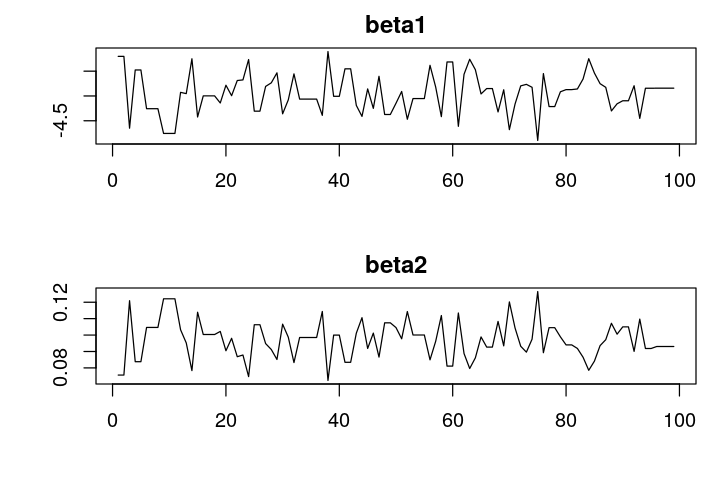Nice! We see that without turning, we get a high acceptance rate. This is the power of IWLS scheme. Let's run the chain longer with thinning to calculate the posterior density.

In :
beta.mcmc <- logit.mcmc(X, y, N=5000, beta0=c(0,0), a, R, thin=10, burnin=1000, seed=1)

 "SUMMARY"
 -------------------------
 "Iterations: 5000"
 "Burn-in: 1000"
 "Thin: 10"
 "Acceptance rate: 0.788"
 -------------------------
 "Mean of beta1: -3.96634292695047"
 "Mean of beta2: 0.0976115122677228"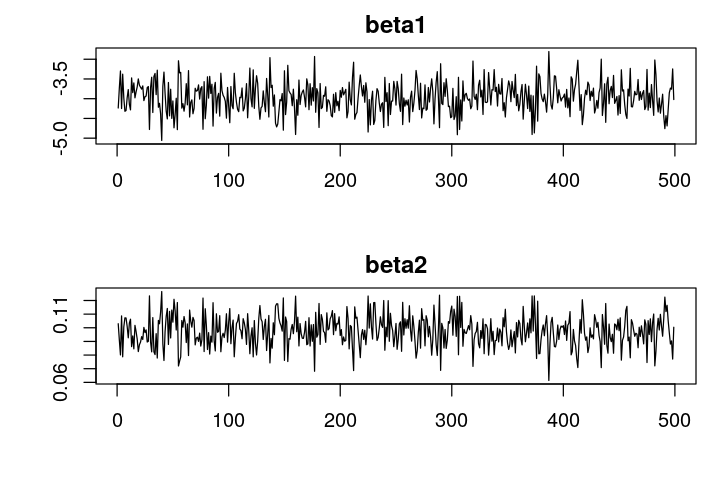The frequentist estimate can be obtained easily using the awesome glm function.

In :
summary(glm(type ~ bmi, family = binomial, data=pima))

Call:
glm(formula = type ~ bmi, family = binomial, data = pima)

Deviance Residuals:
Min       1Q   Median       3Q      Max
-1.9227  -0.8920  -0.6568   1.2559   1.9560

Coefficients:
Estimate Std. Error z value Pr(>|z|)
(Intercept) -4.03681    0.52783  -7.648 2.04e-14 ***
bmi          0.09972    0.01528   6.524 6.84e-11 ***
---
Signif. codes:  0 ‘***’ 0.001 ‘**’ 0.01 ‘*’ 0.05 ‘.’ 0.1 ‘ ’ 1

(Dispersion parameter for binomial family taken to be 1)

Null deviance: 676.79  on 531  degrees of freedom
Residual deviance: 627.46  on 530  degrees of freedom
AIC: 631.46

Number of Fisher Scoring iterations: 4


We see that the Bayesian estimate is very close to the frequentist. Let's plot the logistic curve

$$p(y_i=1 \given \bs{x}_i) = \E (y_i \given \bs{x}_i) = \pi_i = P(\bs{x}_i\tr \bs{\beta}),$$

alongside the original graph. This curve is also the decision function. We will clasify $y_i$ as 1 if $\pi_i \geq 0.5$ and 0 otherwise.

In :
# plotting data
x <- seq(20, 65, 0.1)
pi <- P(0.09972*x - 4.03681)
options(repr.plot.width=3, repr.plot.height=3)
par(mar=c(4, 4, 2, 1))
plot(X[,2], y, xlab='BMI', cex=0.5)
lines(x, pi, col='red')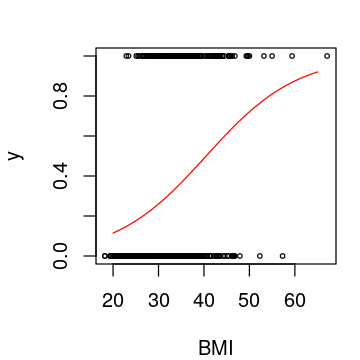## Bivariate Model¶

Finally let's extend our model slightly by adding age as the additional predictor to our model. We can easily fit the model using the glm function.

In :
model <- glm(type ~ bmi + age, family = binomial, data=pima)
summary(model)

Call:
glm(formula = type ~ bmi + age, family = binomial, data = pima)

Deviance Residuals:
Min       1Q   Median       3Q      Max
-2.1748  -0.8322  -0.5262   1.0006   2.2380

Coefficients:
Estimate Std. Error z value Pr(>|z|)
(Intercept) -6.262000   0.672163  -9.316  < 2e-16 ***
bmi          0.103390   0.016066   6.435 1.23e-10 ***
age          0.064147   0.009526   6.734 1.65e-11 ***
---
Signif. codes:  0 ‘***’ 0.001 ‘**’ 0.01 ‘*’ 0.05 ‘.’ 0.1 ‘ ’ 1

(Dispersion parameter for binomial family taken to be 1)

Null deviance: 676.79  on 531  degrees of freedom
Residual deviance: 577.20  on 529  degrees of freedom
AIC: 583.2

Number of Fisher Scoring iterations: 4


In my previous Python posts, I had a lot of fun plotting the decision boundary of a planer classification problem using a meshgrid. We can do the same in R as well!

In :
# define a function that plots decision boundary
decision.plot <- function(model, X, y){
#     Parameters:
#     -----------
#     model = fitted GLM model using the same predictors as data
#     X = new data for prediction (dimension: n x p)
#     y = vector of responses

# obtain minimum and maximum values of predictors
x.min <- min(X[,1]); x.max <- max(X[,1])
y.min <- min(X[,2]); y.max <- max(X[,2])

# building meshgrid
x.grid <- seq(x.min, x.max, (x.max-x.min)/100)
y.grid <- seq(y.min, y.max, (y.max-y.min)/100)
mesh <- expand.grid(x.grid, y.grid) %>% as.data.frame()
names(mesh) <- names(X)

options(repr.plot.width=3, repr.plot.height=3)
par(mar=c(4, 4, 2, 1))

# scatter plotting the data
plot(X[,1], X[,2], col=c('red', 'green')[factor(y)],
xlab=names(X), ylab=names(X), cex=0.5)

# plotting meshgrid
fitted <- predict(model,newdata=mesh,type='response')
fitted <- ifelse(fitted > 0.5, 1, 0)
points(mesh[,1], mesh[,2], col=c('red' ,'green')[factor(fitted)], cex=0.02)
}

In :
data <- pima[c(5,7)]
decision.plot(model, data, y)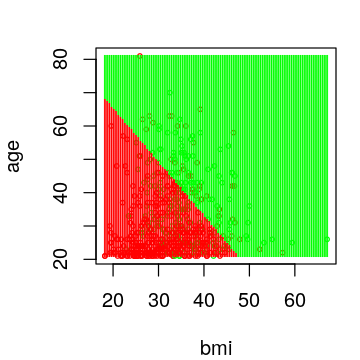Now we are ready to test the Bayesian method for fitting a logistic regression.

In :
# obtain design matrix
X <- cbind(1, pima[,c(5,7)]) %>% as.matrix()

# initialize the chain
beta0 <- c(0,0,0)
a <- c(0,0,0)
R <- 10*diag(3)

In :
beta.mcmc <- logit.mcmc(X, y, N=10000, beta0, a, R, thin=20, burnin=2000)

 "SUMMARY"
 -------------------------
 "Iterations: 10000"
 "Burn-in: 2000"
 "Thin: 20"
 "Acceptance rate: 0.6865"
 -------------------------
 "Mean of beta1: -6.15885991101542"
 "Mean of beta2: 0.101530061037585"
 "Mean of beta3: 0.0629552592846424"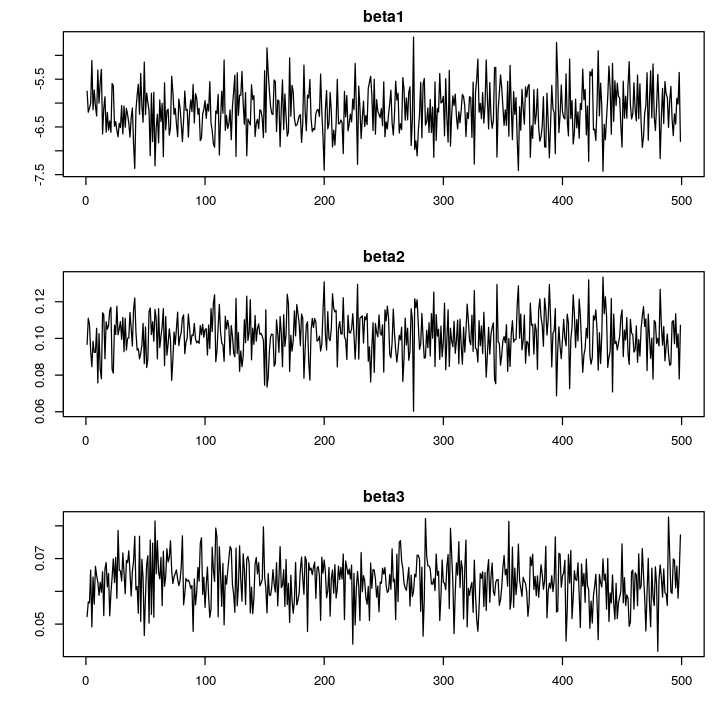So, this is the Bayesian IWLS method for fitting generalized linear models. Stay tuned!